Share

# Balbharati solutions for Balbharati Class 9 Mathematics 2 Geometry chapter 8 - Trigonometry [Latest edition]

Textbook page

#### Chapters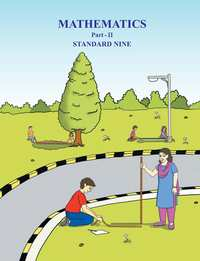## Chapter 8: Trigonometry

Practice Set 8.1Practice Set 8.2Problem Set 8

#### Balbharati solutions for Balbharati Class 9 Mathematics 2 Geometry Chapter 8 TrigonometryExercise Practice Set 8.1 [Page 104]

Practice Set 8.1 | Q 1 | Page 104

In the given Fig, angle R is the right angle of triangle PQR. Write the following ratios.
(i) sin P (ii) cos Q (iii) tan P (iv) tan Q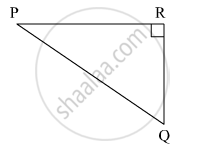Practice Set 8.1 | Q 2 | Page 104

In the right angled triangle XYZ, angleXYZ = 90° and a, b, c are the lengths of the sides as shown in the figure. Write the following ratios,
(i) sin X (ii) tan Z (iii) cos X (iv) tan X.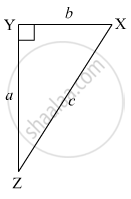Practice Set 8.1 | Q 3 | Page 104
In right angled triangle LMN, angle LMN = 90°, angle L = 50° and ∠ N = 40°, write the following ratios.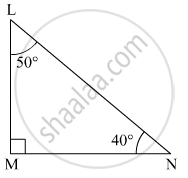(i) sin 50° (ii) cos 50° (iii) tan 40° (iv) cos 40°
Practice Set 8.1 | Q 4 | Page 104

In the given figure, angle PQR = 90° , angle PQS = 90° , angle PRQ = α  and angle QPS = θ   Write the following trigonometric ratios.
(i) sin α, cos α  , tan α
(ii) sin θ , cos θ , tan θ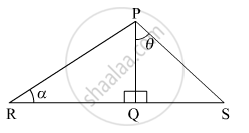#### Balbharati solutions for Balbharati Class 9 Mathematics 2 Geometry Chapter 8 TrigonometryExercise Practice Set 8.2 [Page 112]

Practice Set 8.2 | Q 1 | Page 112

In the following table, a ratio is given in each column. Find the remaining two ratios in the column and complete the table.

 sin θ 11/61 1/2 3/5 Cos θ 35/37 1/sqrt3 tan θ 1 21/20 8/15 1/[2sqrt2]
Practice Set 8.2 | Q 2.1 | Page 112

Find the values of

5 sin 30​° + 3 tan 45°

Practice Set 8.2 | Q 2.2 | Page 112

Find the values of

4/5 tan60° + 3sin2 60°

Practice Set 8.2 | Q 2.3 | Page 112

Find the values of

2sin 30° + cos 0° + 3sin 90°

Practice Set 8.2 | Q 2.4 | Page 112

Find the values of

 [tan 60°]/ [ sin 60° + cos 60°]

Practice Set 8.2 | Q 2.5 | Page 112

Find the values of

cos245 °+ sin2 30°

Practice Set 8.2 | Q 2.6 | Page 112

Find the values of

cos 60°× cos 30° + sin 60°× sin 30°

Practice Set 8.2 | Q 3 | Page 112

If sin θ = 4/5 then find cos θ

Practice Set 8.2 | Q 4 | Page 112

If cos θ = 15/17 then find sin θ

#### Balbharati solutions for Balbharati Class 9 Mathematics 2 Geometry Chapter 8 TrigonometryExercise Problem Set 8 [Page 113]

Problem Set 8 | Q 1.1 | Page 113

Choose the correct alternative answer for following multiple choice question.

Which of the following statements is true ?

• sin θ = cos (90 - θ )

• cos θ = tan ( 90 - θ )

• sin θ = tan (90 - θ )

• tan θ = tan (90 - θ )

Problem Set 8 | Q 1.2 | Page 113

Choose the correct alternative answer for following multiple choice question.

Which of the following is the value of sin 90° ?

• sqrt 3/2

• 0

• 1/2

• 1

Problem Set 8 | Q 1.3 | Page 113

Choose the correct alternative answer for following multiple choice question.

2 tan 45° + cos 45° - sin 45° = ?

• 0

• 1

• 2

• 3

Problem Set 8 | Q 1.4 | Page 113

Choose the correct alternative answer for following multiple choice question.

[cos 28°]/[sin 62°]

• 2

• -1

• 0

• 1

Problem Set 8 | Q 2 | Page 113

In right angled Δ TSU, TS = 5, ∠S = 90°, SU = 12 then find sin T, cos T, tan T. Similarly find sin U, cos U, tan U.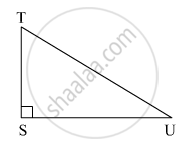Problem Set 8 | Q 3 | Page 113

In right angled ΔYXZ, ∠ X = 90°, XZ = 8 cm, YZ = 17 cm, find sin Y, cos Y, tan Y, sin Z, cos Z, tan Z.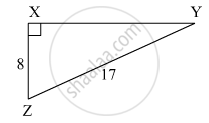Problem Set 8 | Q 4 | Page 113

In right angled Δ LMN , if ∠ N =θ , ∠M = 90°, cos θ =24/25 , find sin θ and tan θ Similarly, find  ( sin2θ) and ( cos2θ).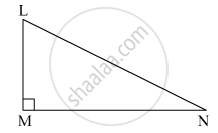Problem Set 8 | Q 5.1 | Page 113

Fill in the blank.
sin20  =  cos ________ °

Problem Set 8 | Q 5.2 | Page 113

Fill in the blank.

tan 30° x tan ______°  = 1

Problem Set 8 | Q 5.3 | Page 113

Fill in the blank.

cos 40° = sin ______°

## Chapter 8: Trigonometry

Practice Set 8.1Practice Set 8.2Problem Set 8## Balbharati solutions for Balbharati Class 9 Mathematics 2 Geometry chapter 8 - Trigonometry

Balbharati solutions for Balbharati Class 9 Mathematics 2 Geometry chapter 8 (Trigonometry) include all questions with solution and detail explanation. This will clear students doubts about any question and improve application skills while preparing for board exams. The detailed, step-by-step solutions will help you understand the concepts better and clear your confusions, if any. Shaalaa.com has the Maharashtra State Board Balbharati Class 9 Mathematics 2 Geometry solutions in a manner that help students grasp basic concepts better and faster.

Further, we at Shaalaa.com provide such solutions so that students can prepare for written exams. Balbharati textbook solutions can be a core help for self-study and acts as a perfect self-help guidance for students.

Concepts covered in Balbharati Class 9 Mathematics 2 Geometry chapter 8 Trigonometry are Introduction to Trigonometry, Terms related to right angled triangle, Trigonometric Ratios of an Acute Angle of a Right-angled Triangle, Important Equation in Trigonometry, Trigonometry Table, Relation among trigonometric ratios.

Using Balbharati Class 9 solutions Trigonometry exercise by students are an easy way to prepare for the exams, as they involve solutions arranged chapter-wise also page wise. The questions involved in Balbharati Solutions are important questions that can be asked in the final exam. Maximum students of Maharashtra State Board Class 9 prefer Balbharati Textbook Solutions to score more in exam.

Get the free view of chapter 8 Trigonometry Class 9 extra questions for Balbharati Class 9 Mathematics 2 Geometry and can use Shaalaa.com to keep it handy for your exam preparation

S